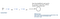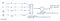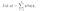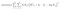# NLP: Word Embedding Algorithms

What are Word Embedding Algorithms and why are they Useful?

Word Embedding algorithms help create more meaningful vector representations for a word in a vocabulary. To train any ML model we need to have inputs as numbers. The input for NLP models is text (words), so we need to have a way to represent this data in numbers. The easiest way to represent a word in a vocabulary, so that we can train language models (for use cases like sentiment classification, language translation) is a one hot vector representation. But using this representation implies that no word is in any way related to another word in the vocabulary. Words like orange, apple, banana are more similar to each other and are used in similar contexts and so having similar representations for these words makes more sense. Word Embedding Algorithms aim to create such representations for words. They capture the relationships between words. We could visualize each number in the vector as corresponding to a feature and hence the embedding is a vector with values for each feature.

Word embeddings let our algorithm understand analogies (like man is to woman as king is to queen). Using word embeddings enable us to build NLP applications with relatively small labeled training sets.

Overview of a few Word Embedding Algorithms

The goal of these algorithms is not to do well on the learning objectives, but to learn good word embeddings.

There are word embeddings readily available online under permissive licenses which have been trained on huge corpuses of data. It’s a good idea to use them instead of training the models from scratch.

WORD2VEC

The main objective of this algorithm is to predict a target word based on a context word. There are different ways to select context words and target word, like:

1. Skip gram model
Predict target words which are randomly chosen words within a window (say +/- 5–10 words) of the input context word.
2. CBow (Continuous bag of words)
Takes the surrounding contexts for a middle word and uses the surrounding words to try to predict the middle word.

Approximate Model Architecture for Skip Gram modelReferenced from Coursera

For understanding the architecture, let’s assume:
1. vocabulary size is 10000 words.
2. ‘glass 34’ means that the word ‘glass’ is the 34th word in the vocabulary
3. 034 is the one hot encoding of the word where the 34th value in the vector is 1 and the size of the vector is vocabulary size i.e 10000.
4. E — a parameter which the model will learn. This is the final Embedding matrix we are trying to build.
5. e34 is the word embedding of the 34th word in the vocabulary. In this case it’s the word embedding of ‘glass’.
6. Softmax layer — Predicts the probabilities for each word in the vocabulary being within the defined window of the input context word. The number of classes is the size of the vocabulary i.e 10000 in our example.

The probability of a particular word in the vocabulary being a target word given a context word for the skip gram model is:

Where Zt = transpose(parameter vector for that target word)*(embedding vector for the context word) + bias and n is the vocabulary size, i.e

Approximate Model Architecture for CBow modelReferenced from Coursera

Similar assumptions/naming conventions as the skip gram model hold for the above architecture as well.

Loss function for the Word2Vec model is the softmax loss function (In the below formula y is a one hot encoded predicted output target vector and y-hat is the one hot encoded correct target vector; n is the vocabulary size).Softmax Loss Function

The problem with the above architectures is that the computation of the Softmax Layer is very expensive and is proportional to the vocabulary size. Generally word embedding algorithms are trained on huge corpuses of data and hence this computation is a bottleneck. Some of the solutions to this problem are:

1. Hierarchical Softmax
Instead of trying to categorize the input into all 10000 categories (vocab size) in one go, it has a binary classifier which first tells if the output is in/ the first x (or a different number) categories or the last 10000-x categories. And then there is another binary classifier which tells if the output is in the first y categories in this sub branch or the last and so on. It mimics a tree structure and eventually we’ll get to know which category the input can be classified(the leaf of the tree) into. Each of the nodes of the tree is a binary classifier. The computational cost of this scales like log(vocabulary size) rather than linear. In practice, this classifier doesn’t use a perfectly balanced or symmetric tree (with equal number of words on each side of the branch), they are developed such that the common words are on the top of the tree and can be reached faster(just a few traversals) and the less common words are buried much deeper in the tree.
2. Negative Sampling
The main architecture change introduced by this idea is replacing the softmax classifier with multiple binary classifiers. The learning objective of the algorithm is changed to: Given a pair of words, predict if this is a context, target pair. For training this model, for each positive sample of a context, target pair we will have ‘k’ negative samples. To generate a positive sample we take a context word and look around a window of +/- 10 words and pick a target word. To generate a negative sample, we take the same context word and pick a word at random from the vocabulary as the target word (under the assumption that the random word picked probably won’t be associated with the context word). ‘k’ is generally in the range of 5–20 for smaller datasets and 2–5 for larger datasets. The model is basically a logistic regression model with the sigmoid activation function applied to the softmax input of the previous architectures. In summary, instead of having one giant 10000 (vocab size) way softmax we have instead turned it into 10000 binary classification problems, each of which is very cheap to compute. And in every iteration we are only going to train (k+1) of the binary classifiers which is relatively cheap to do. There are various heuristic methods to sample negative samples for choosing the target words for the context words and it can be found in the paper.

Modifications to the Word2Vec algorithm have also been made, like Facebook proposed the ‘FastText’ algorithm which is an extension of Word2Vec.

GloVe (Global Vectors for Word Representation)

This is not used as much as the Word2vec model, but it has enthusiasts mainly because of it’s simplicity. Let:

1. Xij = Number of times the word i(target word) appears in the context of j(context word), depending on the definition of context and target words Xij can be equal to Xji.
2. Vocabulary size =n for example: 10000 words

The algorithm’s learning objective is to minimize the difference between transpose(θi)ej (softmax input in the above architectures) and log(Xij). We are trying to measure how related the context and target words are as measured by how often they occur with each other. We are trying to solve for parameters θ and e using gradient descent to minimize the following square cost function:Taken from Coursera

We have an extra weighting term in the beginning of the equation for cases when Xij = 0. It also gives a meaningful amount of computation for less frequent words and makes sure a very huge value isn’t given for frequent words in our corpus. There are various heuristics in choosing this weighting function. One more thing to note here is that, when we look at the equation, the roles of θi and ej are symmetric in terms of optimizing the learning objective. So, we the take final embedding vector of a word as (θi + ej)/2.

References: Coursera